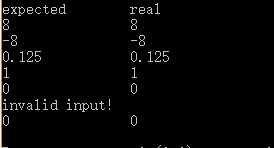• pow函数用于进行求幂运算，只要将底数和指数作为参数传给它，就会返回对应的结果 #include <stdio.h> #include <math.h> int main() { int result = pow(2, 5); printf("%d", result); return ...
pow函数用于进行求幂运算，只要将底数和指数作为参数传给它，就会返回对应的结果


#include <stdio.h>
#include <math.h>

int main()
{
int result = pow(2, 5);
printf("%d", result);
return 0;
}


得到结果 32


展开全文c++
• ## Pow函数

千次阅读 2018-11-29 16:00:18
实现函数double Power(double base, int exponent)，求base的exponent次方。不得使用库函数，同时不需要考虑大数问题。 问题分析 这道题本身并不难，但是要写出完善的代码，需要考虑到各种可能的输入。 1.exponent...
问题描述
实现函数double Power(double base, int exponent)，求base的exponent次方。不得使用库函数，同时不需要考虑大数问题。
问题分析
这道题本身并不难，但是要写出完善的代码，需要考虑到各种可能的输入。
1.exponent为0的情况。
2.exponent 为负数的情况，需要先转为正数计算，再求结果的倒数。
3.base 为0，exponent为负数的情况。因为0不能做分母，这种情况是非法输入，需要进行处理。
代码
#include <iostream>
using namespace std;

double Power(double base, int exponent)
{
if (base == 0.0 && exponent < 0)
{ //基数为0，指数为负，会使0为除数，引发异常
cout << "invalid input!" << endl;
return 0.0;
}
if (exponent == 0)
return 1;

unsigned int absexponent = (unsigned int)exponent;
if (exponent < 0)
absexponent = 0 - exponent;

double result = 1.0;
for (int i = 0; i < absexponent; ++i)
result *= base;

if (exponent < 0)
result = 1.0 / result;

return result;
}
void test()
{
cout << "expected\t" << "real" << endl;
cout << 8 << "\t\t" << Power(2, 3) << endl;
cout << -8 << "\t\t" << Power(-2, 3) << endl;
cout << 0.125 << "\t\t" << Power(2, -3) << endl;
cout << 1 << "\t\t" << Power(2, 0) << endl;
cout << 0 << "\t\t" << Power(0, 4) << endl;
cout << 0 << "\t\t" << Power(0, -4) << endl;
}
int main()
{
test();
return 0;
}


测试结果展开全文• pow函数怎么用？php pow()函数 语法作用：pow()函数的作用是将一个数进行n次方计算后返回语法：pow(X,Y);参数：X表示要做处理的数字Y表示指定n次方中的n数值说明：返回X的Y次方幂，如果参数正确，函数会返回一个数值...
PHP pow函数表示指数表达式。pow函数怎么用？php pow()函数 语法作用：pow()函数的作用是将一个数进行n次方计算后返回语法：pow(X,Y);参数：X表示要做处理的数字Y表示指定n次方中的n数值说明：返回X的Y次方幂，如果参数正确，函数会返回一个数值，如果无法计算，会返回NAN。php pow()函数使用示例：<?php $i = 5;$i = pow($i,3);$j = 6.5;$j = pow($j,4);$k = -5;$k = pow($k,5);$h = -0.5;$h = pow($h,0.6);echo $i."*****".$j."*****".$k."*****".$h;?>输出：125*****1785.0625*****-3125*****NAN本篇文章就是关于PHP pow函数的介绍，希望对需要的朋友有所帮助！
展开全文• python中的pow函数的功能是计算x的y次幂。本篇文章将带大家一起了解一下，pow()函数在Python中的用法。感兴趣的朋友了解一下。以下是 math 模块 pow() 方法的语法:import mathmath.pow( x, y )内置的 pow() 方法pow...
python中的pow函数的功能是计算x的y次幂。本篇文章将带大家一起了解一下，pow()函数在Python中的用法。感兴趣的朋友了解一下。以下是 math 模块 pow() 方法的语法:import mathmath.pow( x, y )内置的 pow() 方法pow(x, y[, z])函数是计算x的y次方，如果z在存在，则再对结果进行取模，其结果等效于pow(x,y) %z注意：pow() 通过内置的方法直接调用，内置方法会把参数作为整型，而 math 模块则会把参数转换为 float。参数：x -- 数值表达式。y -- 数值表达式。z -- 数值表达式。返回值：返回 xy(x的y次方) 的值。以下展示了使用 pow() 方法的实例：一、在命令行中的使用1、pow(x,y):这个是表示x的y次幂。>>> pow(2,4)16>>>2、pow(x,y,z)：这个是表示x的y次幂后除以z的余数>>> pow(2,4,5)1>>>二、在IDE中的使用#!/usr/bin/python# -*- coding: UTF-8 -*-import math   # 导入 math 模块print "math.pow(100, 2) : ", math.pow(100, 2)# 使用内置，查看输出结果区别print "pow(100, 2) : ", pow(100, 2)print "math.pow(100, -2) : ", math.pow(100, -2)print "math.pow(2, 4) : ", math.pow(2, 4)print "math.pow(3, 0) : ", math.pow(3, 0)
展开全文• python中的pow函数的功能是计算x的y次幂。本篇文章将带大家一起了解一下，pow()函数在Python中的用法。感兴趣的朋友了解一下。以下是 math 模块 pow() 方法的语法:import mathmath.pow( x, y )内置的 pow() 方法pow...
• pow()函数用来求baix的y次幂，x、y及函数值都是double型 ，其原型du为：zhidouble pow(double x, double y)。 实例代码如下： #include<stdio.h> #include<...在调用pow函数时，可能导致错误.c语言
• 第一，pow函数的原型 一般来讲，使用一个函数的时候了解他的原型会减少很多不必要的麻烦 原型：extern float pow(float x, float y); 用法：#include <math.h> 功能：计算x的y次幂。 说明：x应大于零，返回幂...
• 幂运算是高更数学的应用学科，是一种关于幂的数学运算。...在python中就有内置函数pow函数表示幂的运算。1、pow()函数Python的内置函数，它计算并返回x的y次方的值。2、语法pow(x, y, z)3、参数x -- 数值表达式...
• 展开全部C语言中的POW函数使用：#include#defineACCURACY100doublefunc1(doublet,intn);doublefunc2(doubleb,intn);doublepow2(doublea,doubleb);intmain(){printf("%lf",pow2(5.21,4.11));return0;}doublepow2...
• 幂运算是高更数学的应用学科，是一种关于幂的数学运算。...在python中就有内置函数pow函数表示幂的运算。1、pow()函数Python的内置函数，它计算并返回x的y次方的值。2、语法pow(x, y, z)3、参数x -- 数值表达式...
• 幂运算是高更数学的应用学科，是一种关于幂的数学运算。...在python中就有内置函数pow函数表示幂的运算。1、pow()函数Python的内置函数，它计算并返回x的y次方的值。2、语法pow(x, y, z)3、参数x -- 数值表达式...
• 幂运算是高更数学的应用学科，是一种关于幂的数学运算。...在python中就有内置函数pow函数表示幂的运算。1、pow()函数Python的内置函数，它计算并返回x的y次方的值。2、语法pow(x, y, z)3、参数x -- 数值表达式...
• 函数名称:pow 函数原型:double pow( double x, double y ); 函数功能:计算x的y次幂 例：z=pow(x,y);...pow函数要用<cmath>头文件。 转载于:https://www.cnblogs.com/lipeiyi520/p/8406489.html...
• pow函数 pow函数是C语言的一个库函数。 函数原型:double pow(double x,double y);功能是计算xy，C语言中没有10x这样的表示。 使用时应注意x，y均为double类型。 pow函数的头文件是#include <math.h>c语言
• 展开全部pow()函数用来求e68a8462616964757a686964616f31333366306466x的y次幂，x、y及函数值都是double型 ，其原型为：double pow(double x, double y)。实例代码如下：#include#includevoid main(){double x = 2, ...
• ## 实现pow函数

千次阅读 2019-06-17 09:24:39
#include <...//pow函数的功能：求第一个参数的第二个参数的次方 double power(double,int);//函数原型,形式参数 int main() { int num1,num2; printf(“请输入；”); scanf("%d%d",&num1,&num...
• ## 自行实现pow函数

千次阅读 2019-04-28 13:47:40
自行实现pow函数 ** 因程序内部存在pow函数，为避免冲突，自定义的函数名改为power #include<stdio.h> #include<stdlib.h> //自行实现pow函数 //求第一个参数的n次幂 //double numl,int num2 ----形式...C语言
• 展开全部pow()函数用来求x的y次幂，x、y及函数值都62616964757a686964616fe58685e5aeb931333366306466是double型 ，其原型为：double pow(double x, double y)。实例代码如下：#include#includevoid main(){double x...
• ## C语言pow函数

千次阅读 2019-09-23 19:48:28
pow(x,y);//其作用是计算x的y次方。x、y及函数值都是double型 例：我要计算2的5次方源代码如下：#include"stdio.h"#include"math.h"main(){long ... /*调用pow函数*/printf("%ld",total);getch();} 转载于:http...
• codeblocks pow函数 出现Not available in current context! pow（x，2*n），x n 的类型都是double 但是怎么默认pow 成int了？ 不是应该是double 怎么处理c
• 第1页共1页c语言pow的用法C语言中pow函数用于计算x的y次幂。下面我们来看看c语言pow的用法。pow函数有以下几种的重载形式：doublepow(doubleX,intY);floatpow(floatX,floatY);floatpow(floatX,intY);longdoublepow......